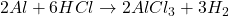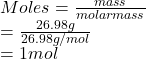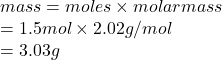## Calculate the mass of hydrogen formed when 26.98 g of aluminum reacts with excess hydrochloric acid according to the following balanced chem

Question

Calculate the mass of hydrogen formed when 26.98 g of aluminum reacts with excess hydrochloric acid according to the following balanced chemical equation: 2 Al + 6 HCl → 2 AlCl3 + 3 H2

in progress 0
6 months 2021-07-23T06:03:25+00:00 1 Answers 21 views 0

## Answers ( )

1. Answer: The mass of hydrogen formed when 26.98 g of aluminum reacts with excess hydrochloric acid according to the given balanced equation is 3.03 g.

Explanation:

The given balanced reaction equation is as follows.Here, the mole ration of Al and hydrogen produced is 2 : 3

As mass of aluminum is given as 26.98 g. So, moles of aluminum (molar mass = 26.98 g/mol) is as follows.So, when 1 mole of Al reacted then 1.5 moles of hydrogen is produced as per the given mole ratio.

Therefore, mass of hydrogen formed is calculated as follows.Thus, we can conclude that the mass of hydrogen formed when 26.98 g of aluminum reacts with excess hydrochloric acid according to the given balanced equation is 3.03 g.